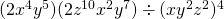Chapter 6: Polynomials

# 6.1 Working with Exponents

Exponents often can be simplified using a few basic properties, since exponents represent repeated multiplication. The basic structure of writing an exponent looks like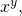where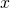is defined as the base and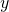is termed its exponent. For this instance,represents the number of times that the variableis multiplied by itself

When looking at numbers to various powers, the following table gives the numeric value of several numbers to various powers.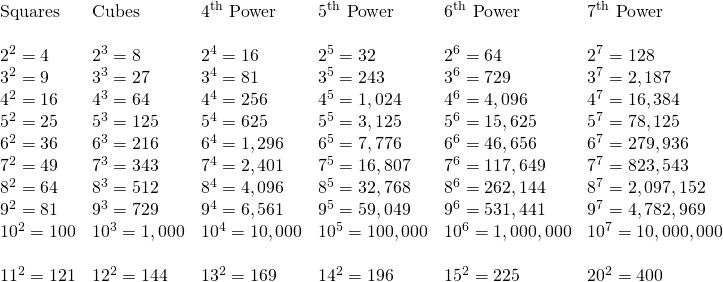For this chart, the expanded forms of the base 2 for multiple exponents is shown: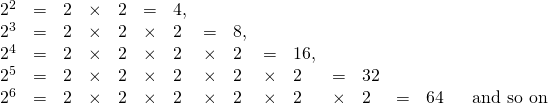Once there is an exponent as a base that is multiplied or divided by itself to the number represented by the exponent, it becomes straightforward to identify a number of rules and properties that can be defined.

The following examples outline a number of these rules.

Example 6.1.1

What is the value of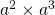?means that you have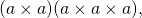which is the same as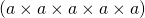or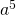This means that, when there is the same base and exponent that is multiplied by the same base with a different exponent, the total exponent value can be found by adding up the exponents.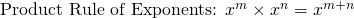Example 6.1.2

What is the value of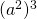?means that you have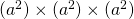,

which is the same as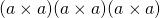or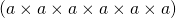,

which equals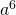When you have some base and exponent where both are multiplied by another exponent, the total exponent value can be found by multiplying the two different exponents together.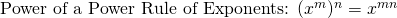Example 6.1.3

What is the value of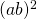?means that you have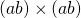,

which is the same as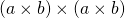or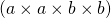,

which equals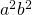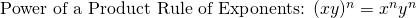Example 6.1.4

What is the value of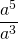?means that you have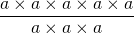, or that you are multiplying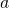by itself five times and dividing it by itself three times.

Multiplying and dividing by the exact same number is a redundant exercise; multiples can be cancelled out prior to doing any multiplying and/or dividing. The easiest way to do this type of a problem is to subtract the exponents, where the exponents in the denominator are being subtracted from the exponents in the numerator. This has the same effect as cancelling any excess or redundant exponents.

For this example, the subtraction looks like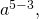leaving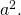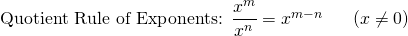Example 6.1.5

What is the value of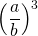?

Expanded, this exponent is the same as: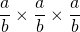Which is the same as: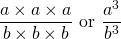One can see that this result is very similar to the power of a product rule of exponents.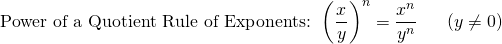# Questions

Simplify the following.

1.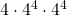2.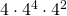3.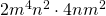4.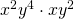5.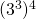6.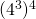7.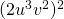8.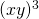9.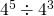10.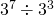11.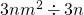12.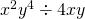13.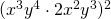14.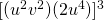15.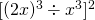16.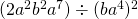17.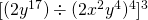18.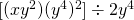19.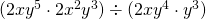20.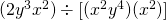21.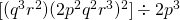22.